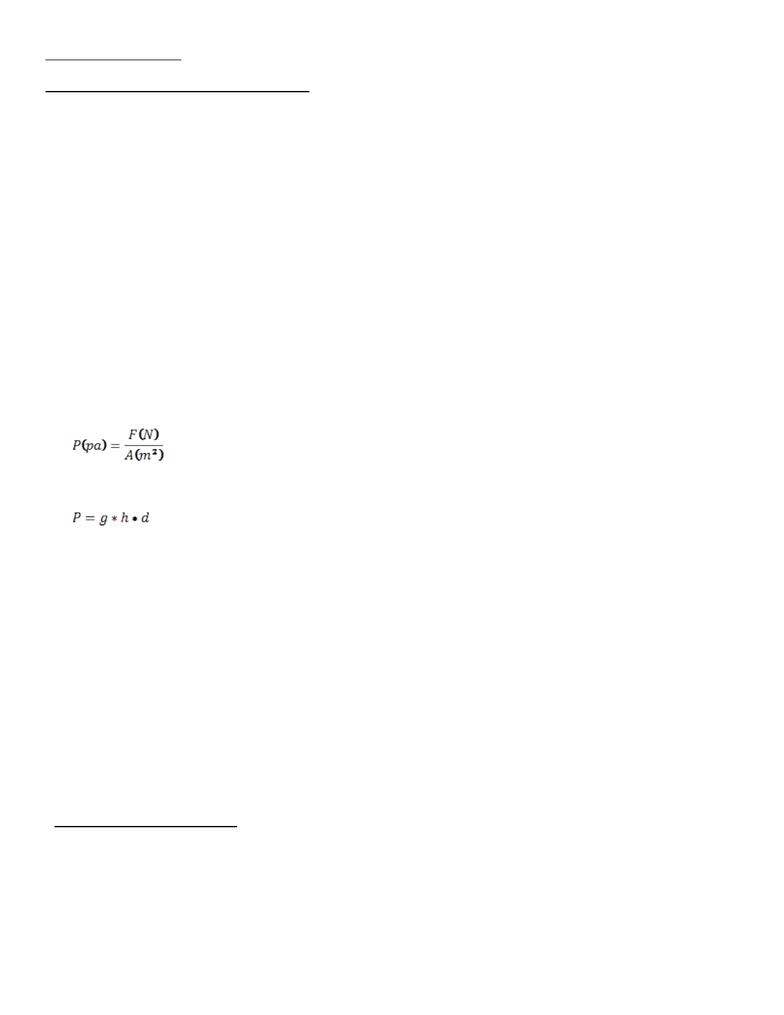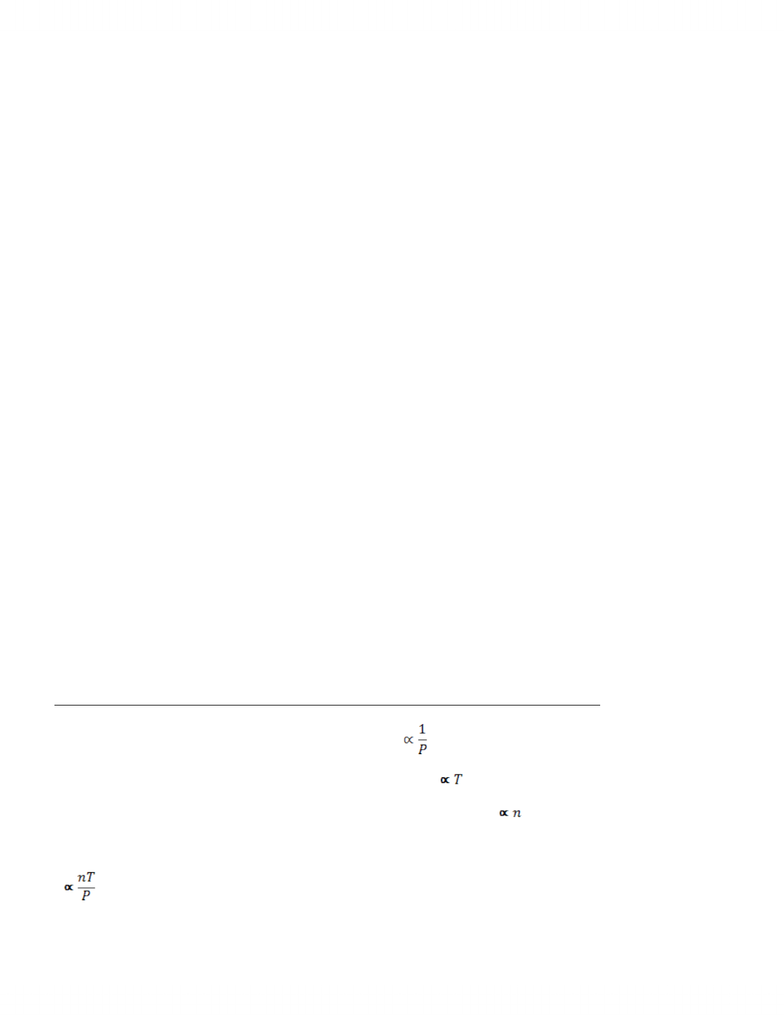Textbook Notes (280,000)
CA (160,000)
UTSC (20,000)
Chemistry (300)
CHMA10H3 (100)
Chapter 6

# CHMA10H3 Chapter Notes - Chapter 6: Adapter Molecule Crk

Department
Chemistry
Course Code
CHMA10H3
Professor
Ann Verner
Chapter
6

This preview shows pages 1-2. to view the full 6 pages of the document.Chapter 6: Gases
6-1:Properties of gases- gas pressure
- Gases expand to fill their containers and assume the shape of their container s
- Diffuse into one another and mix in all proportions
- H2, Methane combustable
4 properties determine the physical behaviour of gases:
1. Amount of gas in moles
2. Volume
3. Pressure
4. Temperature
Concept of pressure:
- Pressure: force per unit area
Liquid pressure:
Barometric pressure:
- Atm ( standard atmosphere) - pressure exerted by a mercury column of exactly 760 mm in
height when the density of mercury is 13.59 g/cm^3
1 atm= 760 mmHg
1 atm = 760 Torr
Standard Condition of Temperature And Pressure:
Manomete=rs:
Pgas=Pbar +changeP
6-2 The Simple Gas Laws:
Boyle’s law: For a fixed amount of gas at the constant temperature , the gas volume is
inversely proportional to the gas pressure

Only pages 1-2 are available for preview. Some parts have been intentionally blurred.-if the weight on the piston is doubled then the pressure is doubled and the volume
decrease to ½ its original.
PV=a ( a constant)
- Gas properties depend on temperate and pressure
- Standard temp = 0 C or 273.15K, standard pressure: 1 atm (STP)
Charles law: The volume of a fixed amount of gas at constant pressure is directly
proportional to the Kelvin (absolute) temperature.
T(K)= t( C) + 273.15
V=bT ( b is a constant)
Standard Temperature and Pressure:
- 0C=273.15K
- 1 atm=760mmHg
- STP ( standard temperature and Pressure)
- Equal volume of different gases compared at the same temp and pressure contains
equal number of molecules
- Law: at a fixed temp and pressure the volume of a gas directly proportional to the
amount of gas ( V prop n, V=c*n)
- 1 mol gas= 22.4L ( at STP)
6.3 combining the gas laws: ideal gas equation and the general gas equation
1. Boyle’s law describes the effect of pressure, V ( PV=a)
2. Charles’s law describes the effect of temperature, V ( V=bT)
3. Avogadro’s Law describes the effect of the amount of gas, V (V= c*n)
Ideal Gas law equation:
V and V= RnT/P